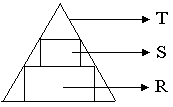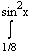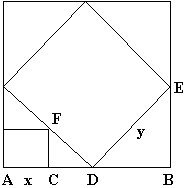# IIT Maths Sample Paper 3

### Calculus

1. Let g(x) be a continuous function such that 0ò1 g(t) dt = 2. Let f(x) = 1/2 0òx (x-t)2 g(t) dt then find f (x) and hence evaluate f "(x).
2. Find area of region bounded by the curve y=[sinx+cosx] between x=0 to x=2p.
3. Solve: [3Öxy + 4y - 7Öy]dx + [4x - 7Öxy + 5Öx]dy = 0.
4. f(x+y) = f(x) + f(y) + 2xy - 1 " x,y. f is differentiable and f (0) = cos a. Prove that f(x) > 0 " x Î R.
5. Show that 0òp q3 ln sin q dq = 3p/2 0òp q2 ln [Ö2 sin q] dq.
6. A function f : R® R satisfies f(x+y) = f(x) + f(y) for all x,y Î R and is continuous throughout the domain. If I1 + I2 + I3 + I4 + I5 = 450, where In = n.0òn f(x) dx. Find f(x).
7.Let T be an acute angled triangle. Inscribe rectangles R & S as shown. Let A(X) denote the area of any polygon X. Find the maximum value of [A(R)+A(S)]/A(T).
8. Evaluate 0òx [x] dx .
9. Let f(x) be a real valued function not identically equal to zero such that f(x+yn)=f(x)+(f(y))n; y is real, n is odd and n >1 and f (0) ³ 0. Find out the value of f (10) and f(5).
10. Evaluate: 0ò11/{ (5+2x-2x2)(1+e(2-4x)) } dx
11. If f(x) is a monotonically increasing function " x Î R, f "(x) > 0 and f -1(x) exists, then prove that å{f -1(xi)/3} < f -1({x1+x2+x3}/3), i=1,2,3
12. Let P(x)= Õ (x-ai), where i=1 to n. and all ais are real. Prove that the derivatives P (x) and P "(x) satisfy the inequality P(x)2 ³ P(x)P"(x) for all real numbers x.
13. Determine the value of 0ò1 xa-1.(ln x)n dx where a Î {2, 3, ...} and n Î N.
14. Evaluate ò sinx dx/[sin(x-p/6).sin(x+p/6)]
15. Discuss the applicability of Rolles theorem to f(x)=log[x2+ab/{(a+b)x}] in the interval [a,b].
16. Let the curve y=f(x) passes through (4,-2) and satisfies the differential equation: y(x+y3)dx = x(y3-x)dy and y=g(x)=sin -1Öt dt +cos -1 Öt dt, 0 £ x £p/2. Find the area of the region bounded by the curves y=f(x), y=g(x) and x=0.
17. Find the polynomial function f(x) of degree 6 which satisfies : Lim(x®0)[1 + f(x)/x3]1/x = e2 and has local maxima at x=1 and local minima at x=0,2.
18. Find all the values of a (a¹ 0) for which: 0òx (t2-8t+13) dt = x sin(a/x). Find that solution.
19.Three squares are shown in the diagram. The largest has side AB of length 1. The others have side AC of length x and side DE of length y. As D moves along AB, find the values of x and y for which x2+y2 is a minimum. What is this minimum?
20. Suppose that the cubic polynomial h(x)=x3 - 3bx2 + 3cx + d has a local maximum A(x1,y1) and a local minimum at B(x2,y2). Prove that the point of inflection of h is at the midpoint of the line segment AB.
21. y = f(x) be a curve passing through (1,1) such that the triangle formed by the coordinate axes and the tangent at any point of the curve lies in the first quadrant and has area 2. Form the differential equation and determine all such possible curves.
22. Let f be a real-valued function defined for all real nos. x such that, for some positive constant a, the equation f(x+a) = 1/2 + Ö(f(x)-(f(x))2) holds for all x. (a) Prove that the function f is periodic. (b) For a=1, give an example of a non-constant function with the required properties.
23. Suppose p, q, r, s are fixed real numbers such that a quadrilateral can be formed with sides p, q, r, and s in clockwise order. Prove that the vertices of the quadrilateral of maximum area lie on a circle.
24. Evaluate 0òµ (x - x3/2 + x5/(2.4) - x7/(2.4.6) + ... )(1 + x2/22 + x4/(2242) + x6/(224262) + ... ) dx.
25. Let f be a twice-differentiable real-valued function satisfying f(x) + f "e;(x) = -xg(x)f '(x), where g(x) ³ 0 for all real x. Prove that |f(x)| is bounded.
26. Let f be a real function on the real line with continuous 3rd derivative. Prove that there exists a point such that f(a).f (a).f "(a).f "(a) ³ 0.
27. The area between the 2 curves defined by y=½x-(x)½ and y=ksin(px), k ³ 1, in the interval 0£ x £1, is found to be equal to 2 sq. units. Find the constant k.Here (x) denotes an integer nearest to x. e.g. (1.3)=1; (1.5)=2; (1.7)=2 and so on.IIT JEE - HomeWebsites of IITJEE SyllabusIIT Question PapersBooks For IITIIT JEE 2008IIT JEE 2007IIT JEE 2006IIT JEE 2005IIT CoursesSeats ReservationEligibility CriteriaAnalysis of IIT JEEIIT AlumniIIT JEE TipsIIT Articles